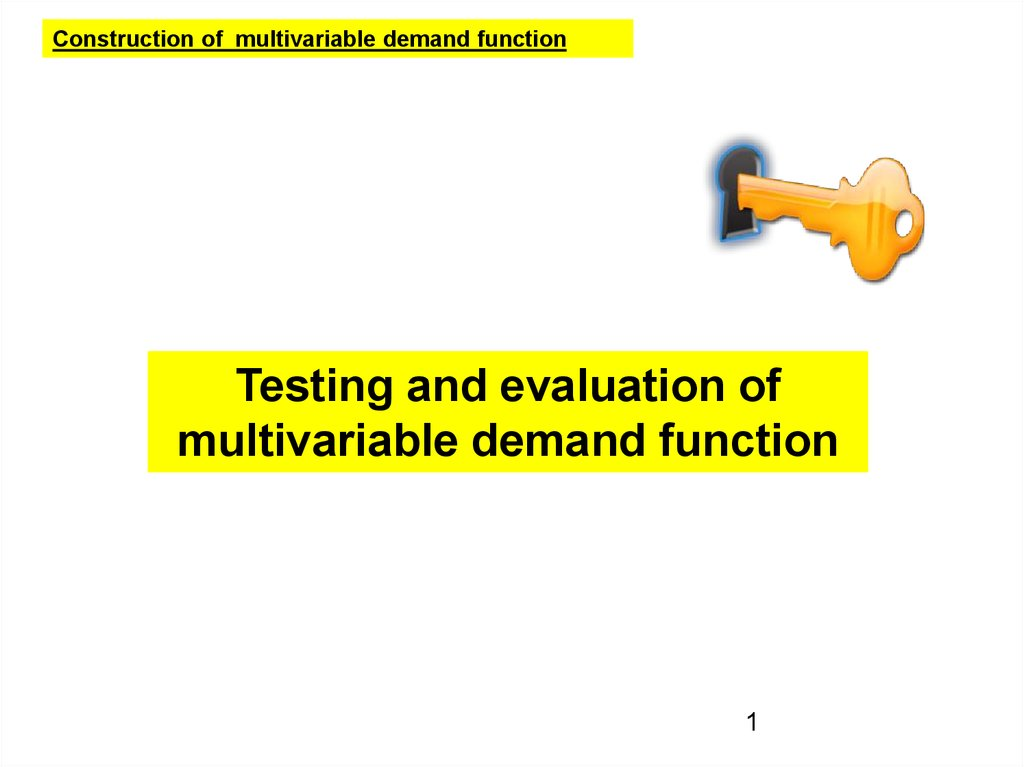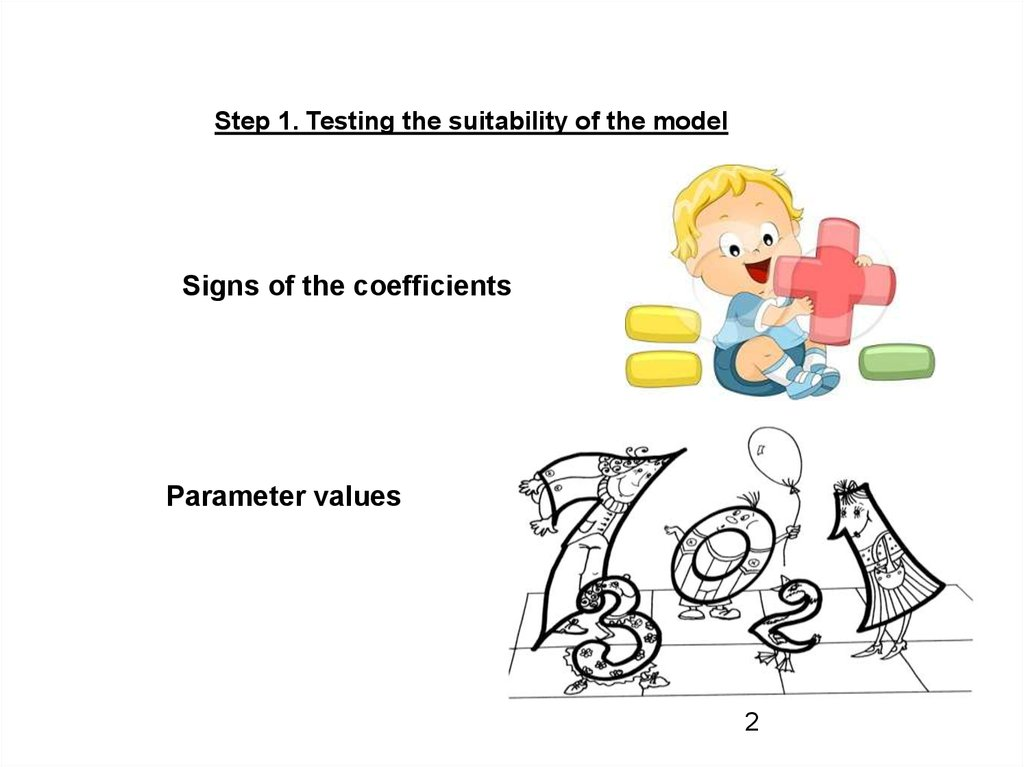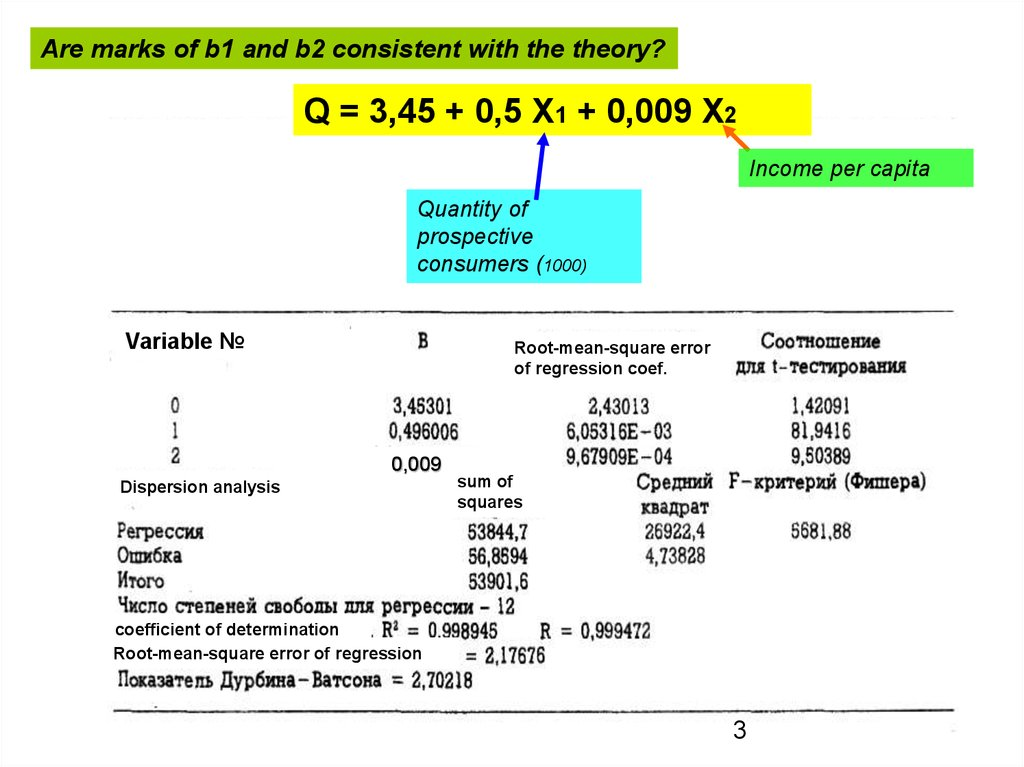# Testing and evaluation of multivariable demand function

## 1.

Construction of multivariable demand function
Testing and evaluation of
multivariable demand function
1

## 2.

Step 1. Testing the suitability of the model
Signs of the coefficients
Parameter values
2

## 3.

Are marks of b1 and b2 consistent with the theory?
Q = 3,45 + 0,5 X1 + 0,009 X2
The sign of the parameter indicates the direction
Income
capita to
of change of the demand variable
withper
respect
changes
in of
the independent variable
Quantity
prospective
consumers (1000)
Demand Variable changes in the same
direction
as the independent
variable
Root-mean-square
error
Variable

The positive
sign:
of regression coef.
The demand variable and the independent
variable are changing in opposite directions
The negative sign:
0,009
Dispersion analysis
sum of
squares
coefficient of determination
Root-mean-square error of regression
3

## 4.

Parameter values
This is parameter validation on
economic sense
The generally accepted limits do not exist, but most
economists subjectively limit values of each parameter
] aggregate demand = a function of prices and disposable income:
Cd = b0 + b1 X1 + b2 X2
] b1 = 2 b2 = 1,3
Do these parameters have
sense?
In accordance with b2, the consumer must spend 1,3 \$
per each additional 1\$ income
4

## 5.

Step 2. Statistical tests and evaluation
Common tests
Plural coefficient of determination, R^2
Corrected plural coefficient of determination, R^2
Root-mean-square error of estimation for the regression
I'm still waiting for the day when I
will need to know the solution of
in real life
5

## 6.

Multiple regression describes the regression plane and the
observed points lie above, below, and on this plane
Step 2. Statistical tests and evaluation
Plural coefficient of determination, R^2
Is a measure of how well the plane described by the regression
equation, satisfies the experimental data
^
^
(
Q
Q
)
(
Q
Q
)
(
Q
Q
)
i
i
i
2
Full variation
=
Explainable variation
2
2
i
+ Unexplained variation
Variation is the sum of the squared deviations of observed values from the regression line
The factor has only mathematical sense and does not determine any
causal relationships
R^2 = Explainable variation /Full variation =
^
(Qi Q)2
(Qi Q)2
6

## 7.

0 < R^2 < 1
R^2 = 0 – there is no relationship between demand and other variables
R^2 = 1 –all changes in demand are explained by simultaneous changes
of the independent variables
0,009
Dispersion analysis
SSR Explainable variation
SSЕ
Unexplained variation
SSТ
Full variation
coefficient of determination
sum of
squares
This means that 99.89 per
cent changes in sales are
explained by changes in the
size of the target population
and per capita income
7

## 8.

Step 2. Statistical tests and evaluation
Corrected plural coefficient of determination, R^2
To get useful results, the number of observations should be sufficient
Pays due attention to the degrees of freedom determined by the
number of observations and number of parameters
k 2
R
R
1
R
n
k
1
2
2
number of observations
The number of independent variables
8
8

## 9.

Acceptable values of
R2 ?
Usually if the number of observations is three or four times
more than the number of independent variables, it is
considered that acceptable value is
R2 0,75
9

## 10.

Step 2. Statistical tests and evaluation
Root-mean-square error of estimation for the regression
Characterizes the dispersion of the observed points from the theoretical
regression line (determines the random scatter of the observed values of
^
Q, relative to the estimated values of Q)
The observed value of the dependent
demand variable in the i-th point
(
Q
Q
)
S
^
i
e
Root-mean-square error of
estimation
2
i
n
k
1Estimated value of the
The number of
independent
variables
dependent demand variable,
calculated for the i-th point on
the regression equation
10

## 11.

0,009
Root-mean-square error of regression
11